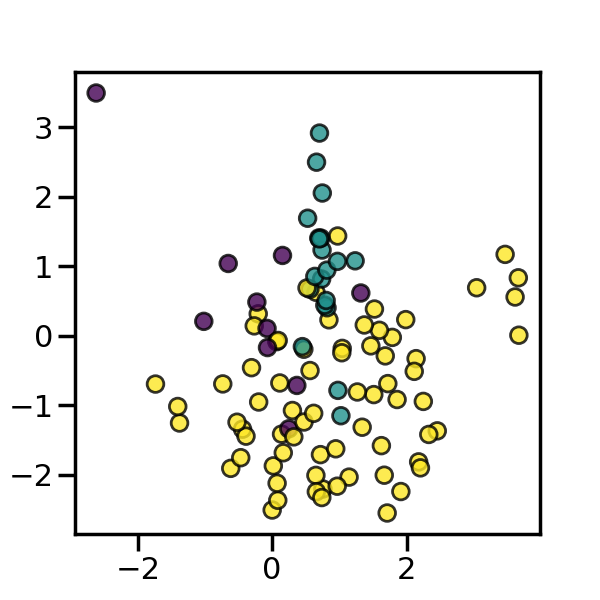# Comparison of the combination of over- and under-sampling algorithms¶

This example shows the effect of applying an under-sampling algorithms after SMOTE over-sampling. In the literature, Tomek’s link and edited nearest neighbours are the two methods which have been used and are available in imbalanced-learn.

```# Authors: Guillaume Lemaitre <g.lemaitre58@gmail.com>

from collections import Counter

import matplotlib.pyplot as plt
import numpy as np

from sklearn.datasets import make_classification
from sklearn.svm import LinearSVC

from imblearn.pipeline import make_pipeline
from imblearn.over_sampling import SMOTE
from imblearn.combine import SMOTEENN, SMOTETomek

print(__doc__)
```

The following function will be used to create toy dataset. It using the `make_classification` from scikit-learn but fixing some parameters.

```def create_dataset(n_samples=1000, weights=(0.01, 0.01, 0.98), n_classes=3,
class_sep=0.8, n_clusters=1):
return make_classification(n_samples=n_samples, n_features=2,
n_informative=2, n_redundant=0, n_repeated=0,
n_classes=n_classes,
n_clusters_per_class=n_clusters,
weights=list(weights),
class_sep=class_sep, random_state=0)
```

The following function will be used to plot the sample space after resampling to illustrate the characteristic of an algorithm.

```def plot_resampling(X, y, sampling, ax):
X_res, y_res = sampling.fit_resample(X, y)
ax.scatter(X_res[:, 0], X_res[:, 1], c=y_res, alpha=0.8, edgecolor='k')
# make nice plotting
ax.spines['top'].set_visible(False)
ax.spines['right'].set_visible(False)
ax.get_xaxis().tick_bottom()
ax.get_yaxis().tick_left()
ax.spines['left'].set_position(('outward', 10))
ax.spines['bottom'].set_position(('outward', 10))
return Counter(y_res)
```

The following function will be used to plot the decision function of a classifier given some data.

```def plot_decision_function(X, y, clf, ax):
plot_step = 0.02
x_min, x_max = X[:, 0].min() - 1, X[:, 0].max() + 1
y_min, y_max = X[:, 1].min() - 1, X[:, 1].max() + 1
xx, yy = np.meshgrid(np.arange(x_min, x_max, plot_step),
np.arange(y_min, y_max, plot_step))

Z = clf.predict(np.c_[xx.ravel(), yy.ravel()])
Z = Z.reshape(xx.shape)
ax.contourf(xx, yy, Z, alpha=0.4)
ax.scatter(X[:, 0], X[:, 1], alpha=0.8, c=y, edgecolor='k')
```

`SMOTE` allows to generate samples. However, this method of over-sampling does not have any knowledge regarding the underlying distribution. Therefore, some noisy samples can be generated, e.g. when the different classes cannot be well separated. Hence, it can be beneficial to apply an under-sampling algorithm to clean the noisy samples. Two methods are usually used in the literature: (i) Tomek’s link and (ii) edited nearest neighbours cleaning methods. Imbalanced-learn provides two ready-to-use samplers `SMOTETomek` and `SMOTEENN`. In general, `SMOTEENN` cleans more noisy data than `SMOTETomek`.

```fig, ((ax1, ax2), (ax3, ax4), (ax5, ax6)) = plt.subplots(3, 2,
figsize=(15, 25))
X, y = create_dataset(n_samples=1000, weights=(0.1, 0.2, 0.7))

ax_arr = ((ax1, ax2), (ax3, ax4), (ax5, ax6))
for ax, sampler in zip(ax_arr, (
SMOTE(random_state=0),
SMOTEENN(random_state=0),
SMOTETomek(random_state=0))):
clf = make_pipeline(sampler, LinearSVC())
clf.fit(X, y)
plot_decision_function(X, y, clf, ax)
ax.set_title('Decision function for {}'.format(
sampler.__class__.__name__))
plot_resampling(X, y, sampler, ax)
ax.set_title('Resampling using {}'.format(
sampler.__class__.__name__))
fig.tight_layout()

plt.show()
```Total running time of the script: ( 0 minutes 3.253 seconds)

Estimated memory usage: 9 MB

Gallery generated by Sphinx-Gallery